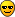# Custom TF1 Sum

Hi all, I have to sum two TF1 objects, custom defined in a .h file.
I didn’t find anything useful in this forum about my problem. Here it is:

[code]TF1* FitFunc1 = new TF1(“Func1”, Func1, 30, 110, 8);
TF1* FitFunc2 = new TF1(“Func2”, Func2, 110, 170, 8);

TF1* FitFunc_tot = new TF1(“Func1+Func2”, “Func1+Func2”, 30, 170, 8);[/code]

the two functions are created in a linked .h file within a function. Am I doing right?

Thank you,
regards

Luca

A similar problem was described in [url]Sum or multiply two TF1 objects

[quote=“ksmith”]A similar problem was described in [url]Sum or multiply two TF1 objects

Ok, but it isn’t working at all… It seems that the FitFunc_tot isn’t the sum of the other two TF1, because I have no fit function on my histogram.

What do you mean it doesn’t work? Can you provide a small working example?

Sure, here’s the example. I add also a few questions about my problem:

I have these 3 functions, the first, the second, and the third is the sum of the 1st and the 2nd. With the 3rd function I have to fit an histogram.

TF1* FunzNo = new TF1(“FunzNo”, FunzNo, 30, 110,;
TF1* FunzFirst = new TF1(“FunzFirst”, FunzFirst, 110, 170,;
TF1* FunzTot = new TF1(“FunzTot”, “FunzNo+FunzFirst”, 30, 170,;

The 1st and the 2nd functions are created in a separate file.h, in particular:

double FunzFirst (double* x, double* params) {
unsigned n_pts1 = nc14_1st;
double q = x;
double de = (2c14_spectrum_endpoint - 2c14_spectrum_startpoint)/(2nc14 -1);
if (n_pts3 % 2 == 0) n_pts1–;
double result = 0;
for (unsigned i = 0; i < n_pts1; i++) {
double energy = de
i;
int factor = ((i % 2) ? 4:2);
if (i == 0 || i + 1 == n_pts1) factor = 1;
result += factor*(c14_energy_spectrum_1st[i]*response_function(q, energy, params));
} result = de/3;
return result
params*params/c14_spectrum_norm + params;
}

The same for the 1st function. So I have to pass to the functions some parameters, from 0 to 8, which are physics stuffs. So I have to set the parameters:

``````    	FunzFirst->SetParameter(0, 0);
FunzFirst->SetParameter(1, 0.463);
FunzFirst->SetParameter(2, 0.01);
FunzFirst->SetParLimits(3, 0, 1);
FunzFirst->SetParameter(4, 0.002);
FunzFirst->SetParameter(5, hist->Integral(30,110));
FunzFirst->FixParameter(6, hist->GetBinWidth(1));
FunzFirst->SetParameter(7, 0.1);
FunzFirst->SetParameter(8, hist->Integral(110,170));
``````

for FunzNo, FunzFirst and FunzTot?

Then I fit with:

hist->Fit(FuncTot, “RV”);

But the result is no function on the histogram.

It is difficult to understand your issue from this partial example. Can you posting a working example?

My first guess would be that you are having issues fitting because you are setting the parameters of FunzFirst and not FunzTot before fitting with FunzTot. Try using the Print command before fitting to see if the initial parameters are reasonable.

```FunzTot->Print(); hist->Fit(FunzTot, "RV");```
Also, you changed the names of the fit pointers from FunzTot to FuncTot

[quote=“ksmith”]It is difficult to understand your issue from this partial example. Can you posting a working example?

My first guess would be that you are having issues fitting because you are setting the parameters of FunzFirst and not FunzTot before fitting with FunzTot. Try using the Print command before fitting to see if the initial parameters are reasonable.

```FunzTot->Print(); hist->Fit(FunzTot, "RV");```
Also, you changed the names of the fit pointers from FunzTot to FuncTot[/quote]

Sure, that’s because the real names of functions are more complicated, I created a fake function for this post named FuncTot (aka FunzTot)
Yes, now I’ll show you the entire code.

I have a similar problem. I’m trying to sum 3 TF1, to get parameters and after that try to evaluate the Integral of gauss. But I get

`Error in <TFormula::Eval>: Formula is invalid and not ready to execute`

This is the code that I’m using

``````TF1* gauss = new TF1("gauss", gaussFit, limInf, limSup, 3);
TF1* noise = new TF1("noise", pol0, limInf, limSup, 1);
TF1* gauss1 = new TF1("gauss1", gaussFit, limInf, limSup, 3);
TF1* funcFit = new TF1("funcFit", "gauss + noise + gauss1", limInf, limSup, 7);

funcFit->SetParName(0,"Amp");
funcFit->SetParName(1,"Mean");
funcFit->SetParName(2,"Std Dev");
funcFit->SetParName(3,"Noise");
funcFit->SetParName(4,"Amp_PileUp");
funcFit->SetParName(5,"Mean_PileUp");
funcFit->SetParName(6,"Std Dev_PileUp");

funcFit->SetParameter(0, 24000);
funcFit->SetParameter(1, 1247);
funcFit->SetParameter(2, 10);
funcFit->SetParameter(3, 10);
funcFit->SetParameter(4, 400);
funcFit->SetParameter(5, 1260);
funcFit->SetParameter(6, 10);

histoSpectre->Fit(funcFit);

cout << "AREA: " << gauss->Integral(1230, 1260) << " +/- " << gauss->IntegralError(1230, 1260) << endl;``````